1592342580

# A Graph Sampling Extension Library for Network

Little Ball of Fur consists of methods that can sample from graph structured data. To put it simply it is a Swiss Army knife for graph sampling tasks. First, it includes a large variety of vertex, edge, and exploration sampling techniques. Second, it provides a unified application public interface which makes the application of sampling algorithms trivial for end-users. Implemented methods cover a wide range of networking (Networking, INFOCOM, SIGCOMM) and data mining (KDD, TKDD, ICDE) conferences, workshops, and pieces from prominent journals.
If you find Little Ball of Fur and the new datasets useful in your research, please consider citing the following paper:

#machine learning

## Buddha Community1595691960

## How Graph Convolutional Networks (GCN) Work

In this post, we’re gonna take a close look at one of the well-known Graph neural networks named GCN. First, we’ll get the intuition to see how it works, then we’ll go deeper into the maths behind it.

## Why Graphs?

Many problems are graphs in true nature. In our world, we see many data are graphs, such as molecules, social networks, and paper citations networks.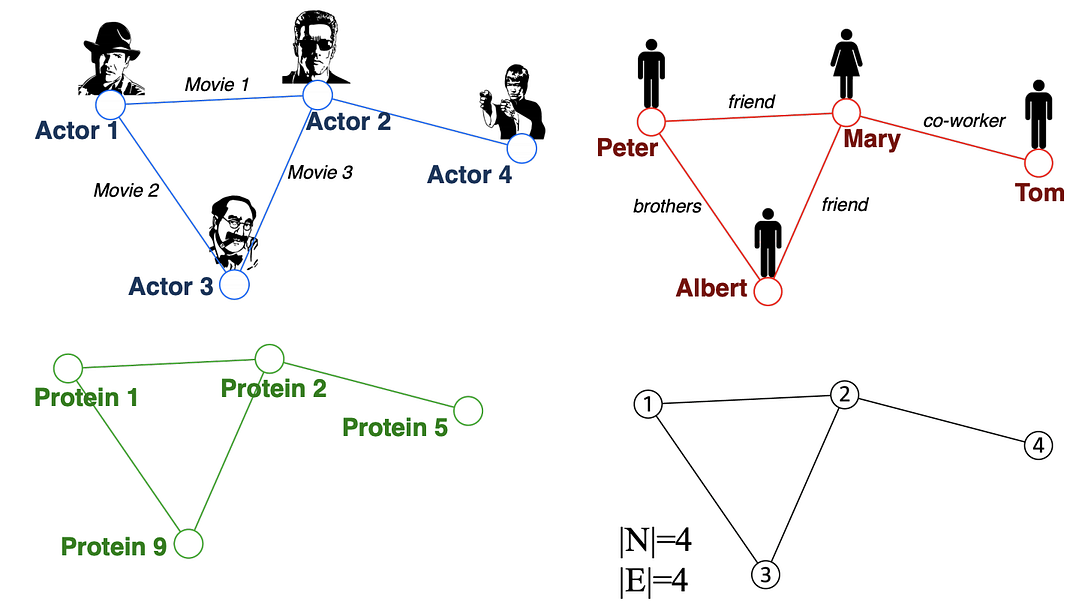Examples of graphs. (Picture from )

• Node classification: Predict a type of a given node
• Community detection: Identify densely linked clusters of nodes
• Network similarity: How similar are two (sub)networks

## Machine Learning Lifecycle

In the graph, we have node features (the data of nodes) and the structure of the graph (how nodes are connected).

For the former, we can easily get the data from each node. But when it comes to the structure, it is not trivial to extract useful information from it. For example, if 2 nodes are close to one another, should we treat them differently to other pairs? How about high and low degree nodes? In fact, each specific task can consume a lot of time and effort just for Feature Engineering, i.e., to distill the structure into our features.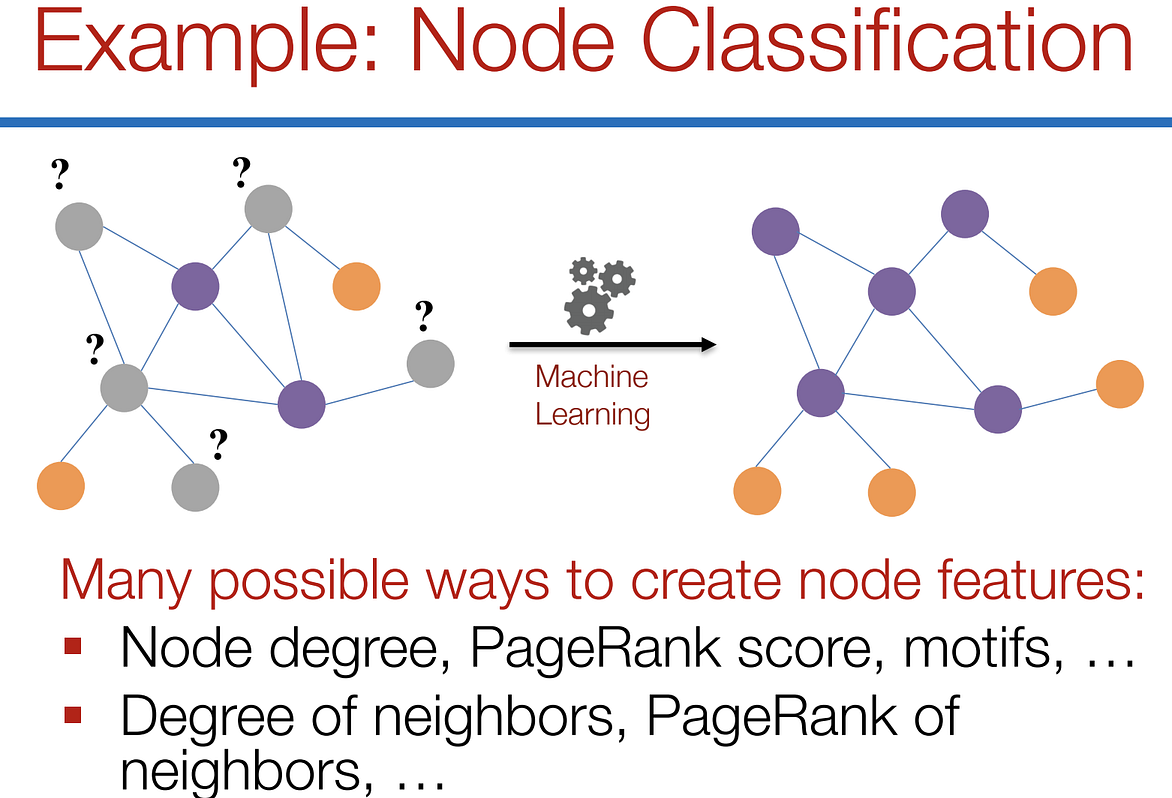Feature engineering on graphs. (Picture from )

It would be much better to somehow get both the node features and the structure as the input, and let the machine to figure out what information is useful by itself.

That’s why we need Graph Representation Learning.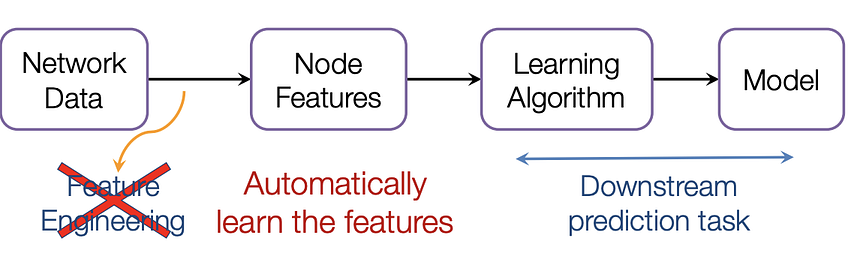We want the graph can learn the “feature engineering” by itself. (Picture from )

# Graph Convolutional Networks (GCNs)

Paper: Semi-supervised Classification with Graph Convolutional Networks(2017) 

GCN is a type of convolutional neural network that can work directly on graphs and take advantage of their structural information.

it solves the problem of classifying nodes (such as documents) in a graph (such as a citation network), where labels are only available for a small subset of nodes (semi-supervised learning).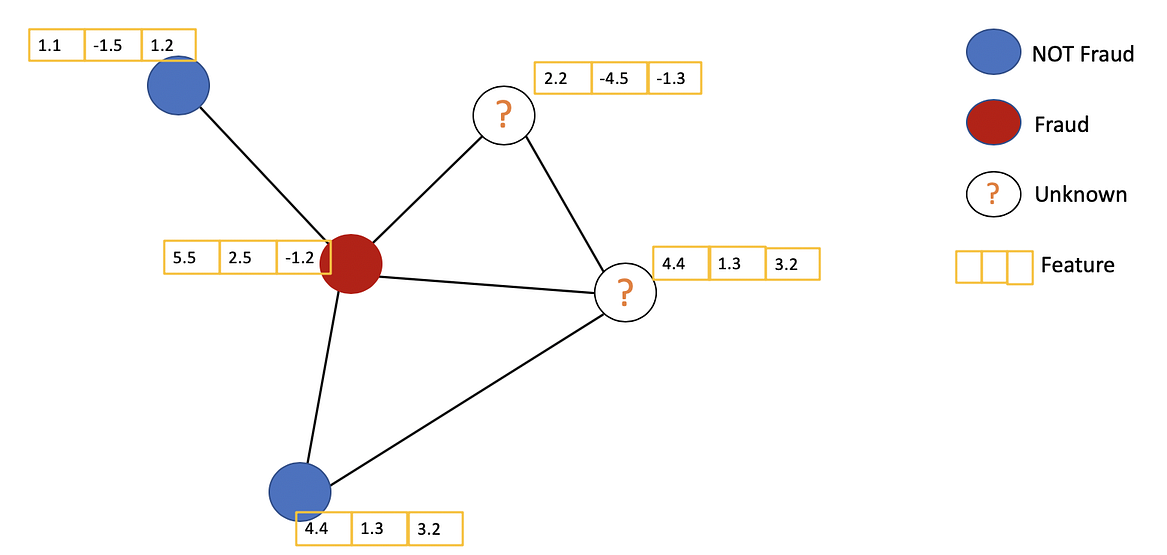Example of Semi-supervised learning on Graphs. Some nodes dont have labels (unknown nodes).

#graph-neural-networks #graph-convolution-network #deep-learning #neural-networks1602838800

## SimGNN: Similarity Computation via Graph Neural Networks

This post will summarize the paper SimGNN which aims for fast graph similarity computation. Graphs are structures that are used to link different entities that we call nodes using relationships called edges. Graphs exist everywhere from bonds between the atoms to friends on Facebook, all these scenarios can be represented as a graph. One of the fundamental graph problems includes finding similarity between graphs. The similarity between graphs can be defined using these metrics :

1. Graph Edit Distance
2. Maximum Common Subgraph

However, currently available algorithms that are used to calculate these metrics have high complexities and it is not yet possible to compute exact GED using these for graphs having more than 16 nodes.

Some ways to compute these metrics are :

1. Pruning verification Framework
2. Approximating the GED in fast and heuristic ways

SimGNN follows another approach to tackle this problem i.e turning similarity computation problem into a learning problem.

Before getting into how SimGNN works, we must know the requirements to be satisfied by this model. It includes :

1. Representation Invariant: Different representations of the same graph should give the same results.
2. **Inductive: **Should be able to predict results for unseen graphs.
3. Learnable: Must work on different similarity metrics like GED and MCS

**SimGNN Approach: **To achieve the above-stated requirements, SimGNN uses two strategies

1. Design Learnable Embedding Function: This maps the graph into an embedding vector, which provides a global summary of a graph. Here, some nodes of importance are selected and used for embedding computation. (less time complexity)
2. Pair-wise node comparison: The above embedding are too coarse, thus further compute the pairwise similarity scores between nodes from the two graphs, from which the histogram features are extracted and combined with the graph level information. (this is a time-consuming strategy)

#graph-edit-distance #machine-learning #graph-neural-networks #graph-convolution-network1596401640

## Do Graph Databases Scale?

Graph Databases are a great solution for many modern use cases: Fraud Detection, Knowledge Graphs, Asset Management, Recommendation Engines, IoT, Permission Management … you name it.

All such projects benefit from a database technology capable of analyzing highly connected data points and their relations fast – Graph databases are designed for these tasks.

But the nature of graph data poses challenges when it comes to buzzword alert scalability. So why is this, and are graph databases capable of scaling? Let’s see…

In the following, we will define what we mean by scaling, take a closer look at two challenges potentially hindering scaling with graph databases, and discuss solutions currently available.

## What Is the “Scalability of Graph Databases”?

Let’s quickly define what we mean here by scaling, as it is not “just” putting more data on one machine or throwing it on various ones. What you want when working with large or growing datasets is also an acceptable performance of your queries.

So the real question here is: Are graph databases able to provide acceptable performance when data sets grow on a single machine or even exceed its capabilities?

You might ask why this is a question in the first place. If so, please read the quick recap about graph databases below. If you are already aware of the issues like supernodes and network hops, please skip the quick recap.

### Quick Recap About Graph Databases

In a nutshell, graph databases store schema-free objects (vertices or nodes) where arbitrary data can be stored (properties) and relations between the objects (edges). Edges typically have a direction pointing from one object to another. Vertices and edges form a network of data points which is called a “graph”.

In discrete mathematics, a graph is defined as a set of vertices and edges. In computing, it is considered an abstract data type that is good at representing connections or relations – unlike the tabular data structures of relational database systems, which are ironically very limited in expressing relations.

As mentioned above, graphs consist of nodes aka vertices (V) connected by relations aka edges (E).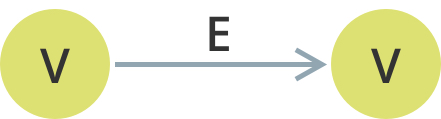Vertices can have an arbitrary amount of edges and form paths of arbitrary depth (length of a path).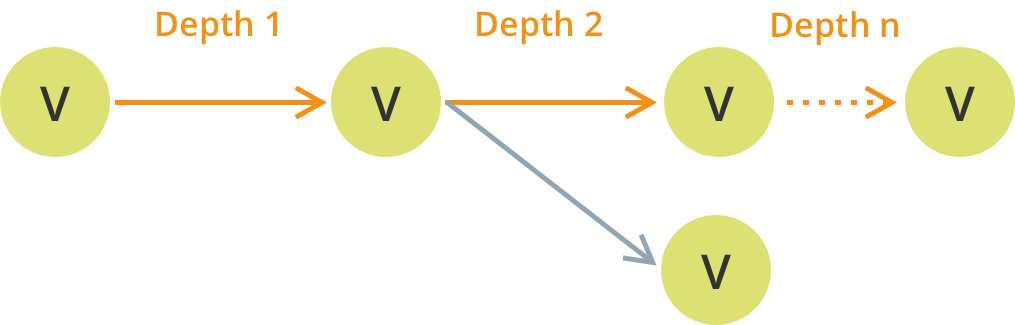A financial transaction use case from one bank account to the other could be modeled as a graph as well and look like the schema below. Here you might define bank accounts as nodes and bank transactions among other relationships as edges.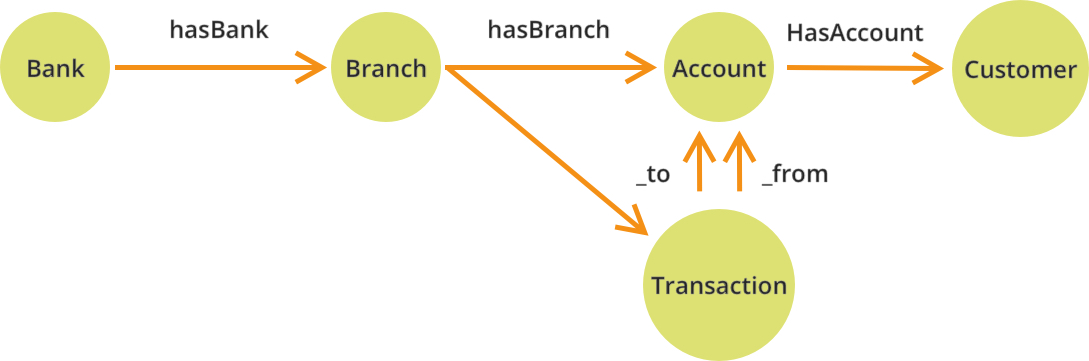Storing accounts and transactions in this way will enable us to traverse the created graph is unknown or varying depth. Composing and running such queries in relational databases tends to be a complex endeavor. (Sidenote: With a multi-model database, we could also model the relationship between banks and their branches as a simple relation using joins to query). If you want to learn more about graph databases, you can check out this free course.

Graph databases provide various algorithms to query stored data and analyze relationships. Algorithms may include traversals, pattern matching, shortest path, or distributed graph processing like community detection, connected components, or centrality.

Most algorithms have one thing in common which is also the nature of the supernode and network hop problem. Algorithms traverse from one node via an edge to another node.

After this quick recap, let’s dive into the challenges. First off: celebrities.

## Always These Celebrities

As described above, vertices or nodes can have an arbitrary amount of edges. A classic example of the supernode problem is celebrities in a social network. A supernode is a node in a graph dataset with unusually high amounts of incoming or outgoing edges.

For instance, Sir Patrick Stewart’s Twitter account has currently over 3.4 million followers.If we now model accounts and tweets as a graph and traverse through the dataset, we might have to traverse over Patrick Stewart’s account and the traversal algorithms would have to analyze all 3.4m edges pointing to Mr. Steward’s account. This will increase the query execution time significantly and may even exceed acceptable limits. Similar problems can be found in e.g. Fraud Detection (accounts with many transactions), Network Management (large IP hubs), and other cases.

Supernodes are an inherent problem of graphs and pose challenges for all graph databases. But there are two options to minimize the impact of supernodes.

### Option 1: Splitting Up Supernodes

To be more precise, we can duplicate the node “Patrick Stewart” and split up a large number of edges by a certain attribute like the country the followers are from or some other form of grouping. Thereby, we minimize the impact of the supernode on traversal performance in case we can make use of these groupings in the queries we want to perform.

### Option 2: Vertex-Centric Indexes

vertex-centric index stores information of an edge together with information about the node.

To stay in the example of Patrick Stewart’s Twitter account, depending on the use case, we could use

• date/time information about when someone started to follow
• the country the follower is from
• follower counts of the follower
• etc.

All these attributes might provide the selectivity we need to effectively use a vertex-centric index.

The query engine can then use the index to lower the number of linear lookups needed to perform the traversal. The same approach can be used e.g. Fraud Detection. Here financial transactions are the edges and we could use the transaction dates or amounts for achieving high selectivity.

There are cases where using the options described above is not suitable and one has to live with some degree of performance degradation when traversing over supernodes. Yet, in most cases, there are options to optimize performance. But there is another challenge, which has not been solved by most graph databases.

#graph database #graph analytics #arangodb #fraud detection #knowledge graph #network management #network latency1594478340

## How to Explain Graph Neural Network — GNNExplainer

Graph Neural Network(GNN) is a type of neural network that can be directly applied to graph-structured data. My previous post gave a brief introduction on GNN. Readers may be directed to this post for more details.

Many research works have shown GNN’s power for understanding graphs, but the way how and why GNN works still remains a mystery for most people. Unlike CNN, where we can extract activation of each layer to visualize the decisions of the network, in GNN it is hard to get a meaningful explanation of what features the network has learnt. Why does GNN determine a node is class A instead of class B? Why does GNN determine a graph is a chemical or molecule? It seems like GNN sees some useful structural information and determines are made upon these observations. But now the problem is, what observations does GNN see?

## What is GNNExplainer?

GNNExplainer is introduced in this paper.

Briefly speaking, it is trying to build a network to learn what a GNN has learnt.

The main principle of GNNExplainer is by reducing redundant information in a graph which does not directly impact the decisions. To explain a graph, we want to know what are the crucial features or structures in the graph that affect the decisions of a neural network. If a feature is important, then the prediction should be altered largely by removing or replacing this feature with something else. On the other hand, if removing or altering a feature does not affect the prediction outcome, the feature is considered not essential and thus should not be included in the explanation for a graph.

## How does it work?

The primary objective for GNNExplainer is to generate a minimal graph that explains the decision for a node or a graph. To achieve this goal, the problem can be defined as finding a subgraph in the computation graph, that minimizes the difference in the prediction scores using the whole computation graph and the minimal graph. In the paper, this process is formulated as maximizing the mutual information(MI) between the minimal graph Gs and the computation graph G: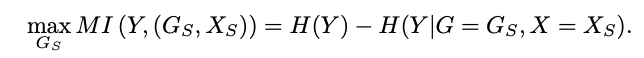Besides, there is a secondary objective: the graph needs to be minimal. Though it was also mentioned in the first objective, we need to have a method to formulate this objective as well. The paper addresses it by adding a loss for the number of edges. Therefore, the loss for GNNExplainer is literally the combination of prediction loss and edge size loss.

#graph #graph-neural-networks #graph-theory #pattern-recognition #machine-learning1594474140

## Benchmarking Graph Neural Networks

Graph Neural Networks (GNNs) are widely used today in diverse applications of social sciences, knowledge graphs, chemistry, physics, neuroscience, etc., and accordingly there has been a great surge of interest and growth in the number of papers in the literature.

However, it has been increasingly difficult to gauge the effectiveness of new models and validate new ideas that generalize universally to larger and complex datasets in the absence of a standard and widely-adopted benchmark.

To address this paramount concern existing in graph learning research, we develop an open-source, easy-to-use and reproducible benchmarking framework with a rigorous experimental protocol that is representative of the categorical advances in GNNs.

This post outlines the issues in the GNN literature suggesting the need of a benchmark, the framework proposed in the paper, the broad classes of widely used and powerful GNNs benchmarked and the insights learnt from the extensive experiments._

# Why benchmark?

In any core research or application area in deep learning, a benchmark helps to identify and quantify what types of architecturesprinciples, or mechanisms are universal and generalizable to real-world tasks and large datasets. Particularly, the recent revolution in this AI field is often credited, to a possibly large extent, to be triggered by the large-scale benchmark image dataset, ImageNet. (Obviously, other driving factors include increase in the volume of research, more datasets, compute, wide-adoptance, etc.)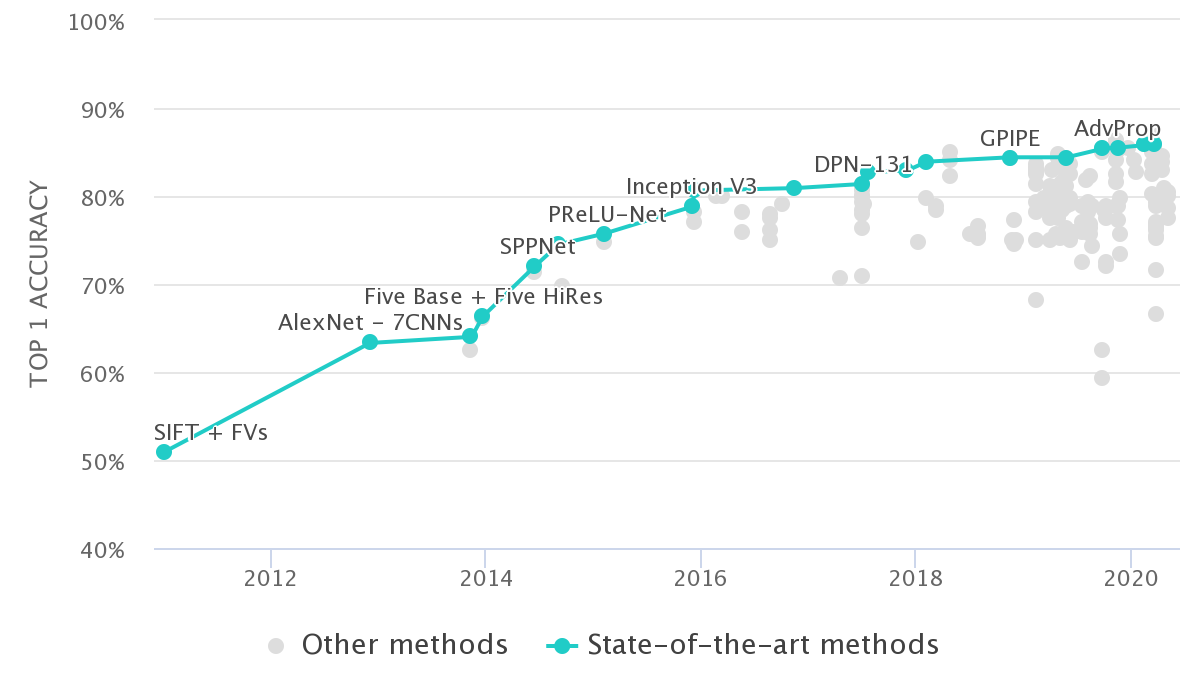Fig 1: ImageNet Classification Leaderboard from paperswithcode.com

Benchmarking has been proved to be beneficial for driving progress, identifying essential ideas, and solving domain-related problems in many sub-fields of science. This project was conceived with this fundamental motivation.

# Need of a benchmarking framework for GNNs

## a. Datasets:

Many of the widely cited papers in the GNN literature contain experiments that are evaluated on small graph datasets which have only a few hundreds (or, thousand) of graphs.Fig 2: Statistics of the widely used TU datasets. Source Errica et al., 2020

Take for example, the ENZYMES dataset, which is almost seen in every work on a GNN for classification task. If one uses a random 10-fold cross validation (in most papers), the test set would have 60 graphs (i.e. 10% of 600 total graphs). That would mean a correct classification (or, alternatively a misclassification) would change 1.67% of test accuracy score. A couple of samples could determine a 3.33% difference in performance measure, which is usually a significant gain score stated when one validates a new idea in literature. You see there, the number of samples is unreliable to concretely acknowledge the advances.¹

Our experiments, too, show that the standard deviation of performance on such datasets is large, making it difficult to make substantial conclusions on a research idea. Moreover, most GNNs perform statistically the same on these datasets. The quality of these datasets also leads one to question if you should use them while validating ideas on GNNs. On several of these datasets, simpler models, sometimes, perform as good, or even beats GNNs.

Consequently, it has become difficult to differentiate complexsimple and graph-agnostic architectures for graph machine learning.

## b. Consistent experimental protocol:

Several papers in the GNN literature do not have consensus on a unifying and robust experimental setting which leads to discussing the inconsistencies and re-evaluating several papers’ experiments.

For a couple of examples to highlight here, Ying et al., 2018 performed training on 10-fold split data for a fixed number of epochs and reported the performance of the epoch which has the “highest average validation accuracy across the splits at any epoch” whereas Lee et al., 2019 used an “early stopping criterion” by monitoring the epoch-wise validation loss and report “average test accuracy at last epoch” over 10-fold split.

Now, if we extract results of both these papers to put together in the same table and claim that the model with the highest performance score is the promising of all, can we get convinced that the comparison is fair?

There are other issues related to hyperparamter selection, comparison in an unfair budgets of trainable parameters, use of different train-validation-test splits, etc.

The existence of such problems pushed us to develop a GNN benchmarking framework which standardizes GNN research and help researchers make more meaningful advances.

#benchmarking #graph #graph-deep-learning #graph-neural-networks #deep-learning